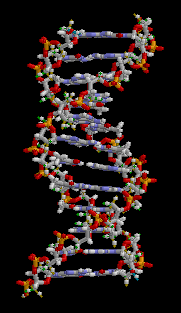• 汇集公众科学智慧交流科学思想见解
• 点燃科学智慧火花构建互动交流平台## 脱氧核糖核酸与哥德巴赫猜想结构及密码学

20世纪发现5大模型（构造）1.生物学的DNA模型。2.地球物理学的板块模型。3.天体物理学的宇宙大爆炸模型。4.量子物理学的夸克模型。5.数学中的素数模型及哥德巴赫猜想结构。

那么，这五个模型有没有关系呢？

这种叫做DNA的脱氧核糖核酸是一种双螺旋结构，是一种由四个碱基对应的模式。与素数模型应用于哥德巴赫猜想有相似的东西：DNA的与两股脱氧核糖核酸长链上的碱基以氢键相互吸引，使双螺旋形态得以维持。

1，如果n是合数，则它有一个因子d满足1 < d ≤。,

2，若自然数n不能被不大于任何素数整除，则n是一个素数（【代数学词典】259页，上海教育出版社）。.....(1).........（2）$N=p_{{1}}m_{{1}}+d_{{1}}$=$p_{{1}}m_{{1}}+d_{{1}}$=...=$p_{{k}}m_{{k}}+d_{{k}}$....(4)

其中，$d_{i}$=0， 1，2， ,,...，,$p_{i-1}$$p_{1}$$p_{2}$，,...,，$p_{k}$.表示前面k个顺序素数2，3，5，....。$\frac{P_{K^{2}}}{~{}2~{}}$ < N <$\frac{P_{K+1}^{2}}{~{}2~{}}$

现在问，是否存在X,.....（5）$f_{i}$$d_{i}$,$f_{i}$$p_{{i}}-d_{{i}}$,

（5）式的同余形式：$X \equiv f_{1} \pmod{p_{1}}$,$X\equiv f_{{2}}{\pmod {p_{{2}}}}$,...,$X\equiv f_{{k}}{\pmod {p_{{k}}}}$....(6)$\frac{5^{2}}{~{}2~{}}$ < 20 <$\frac{7^{2}}{~{}2~{}}$$d_{{1}}=0$,；$d_{{2}}=2$,；$d_{{3}}=0$.。

 构造x21 27 3 9$f_{i}$≠$d_{i}$,$f_{i}$≠$p_{{i}}-d_{{i}}$,，，.，，.，，.，，.

DNA 与图灵机，密码学